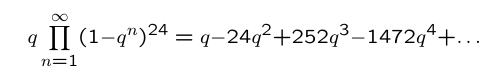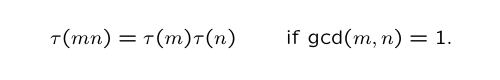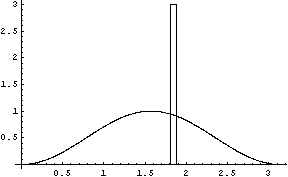1This page was created by Julian Rosen and Ralf Schmidt at the University of Oklahoma Mathematics Department. Our goal is to give an easy introduction to the Sato-Tate Conjecture in the theory of modular forms and elliptic curves.

The Sato-Tate Conjecture is a statement about the statistical distribution of certain sequences of numbers. As an example, consider the following formal product in the variable q:If we multiply everything out, we get a formal power series:The coefficient of qn in this power series is traditionally called τ(n), and the function that maps n to τ(n) is called the Ramanujan τ-Function.
 n τ(n) 1 1 2 -24 3 252 4 -1472 5 4830 6 -6048 7 -16744 8 84480 9 -113643 10 -115920 997 -21400415987399554
The numbers τ(n) have several remarkable properties, one of which isAnother property isfor p a prime number and r a positive integer. It is therefore enough to know the values τ(p) for prime numbers p. Ramanujan had conjectured in 1917, and Deligne proved in 1970, that
 p |τ(p)|p-11/2 2 0.53033 3 0.598734 5 0.691213 7 0.376548 11 1.00087 13 0.431561 17 1.17965 19 0.987803 23 0.603975 29 1.16251 997 0.688016for prime numbers p. Therefore, there exists a unique angle &phip, the Frobenius angle, between 0 and π such thatThe Sato-Tate conjecture asserts that the angles φp are distributed according to the functionThe following displays allow you to explore this conjecture. The left graph below shows the function (2/π)sin2(φ) and the location of the first Satake parameter. You can view the distribution of more parameters (up to 1000) by clicking on the buttons. On the right side we have an animation running through these first 1000 graphs.
Sato-Tate Graphs for the Ramanujan τ-Function (1000 Parameters, Step 1)The following display is similar, except that we are working with the first 5000 primes and a step size of 50.
Sato-Tate Graphs for the Ramanujan τ-Function (5000 Parameters, Step 50)There is more - go to page 2.
Or click on the red box on top of the page in order to navigate.